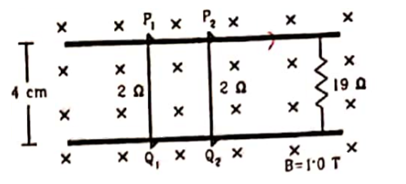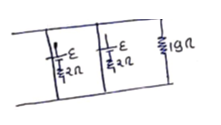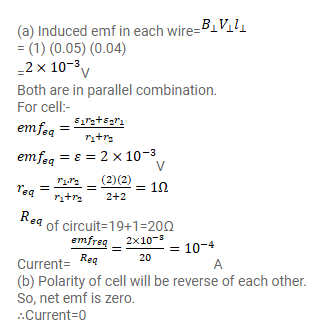# Consider the situation given in the figure.

Question:

Consider the situation given in the figure. The wires $P_{1} Q_{1}$ and $P_{2} Q_{2}$ are made to slide on the rails with the same speed 5 $\mathrm{cm} / \mathrm{s}$. Find the electric current in the $19 \Omega$ resistor if (a) both the wires move towards right and (b) if $P_{1} Q_{1}$ moves towards left but $P_{2} Q_{2}$ moves towards right.Solution: